Published: 17 November 2023

# Thermal performance investigation of porous fins with convection and radiation under the influence of magnetic field using optimal homotopy asymptotic method

M. G. Sobamowo1
H. Berrehal2
A. B. Ajayi3
O. K. Onanuga4
R. O. Fawumi5
1, 3Department of Mechanical Engineering, University of Lagos, Lagos, Nigeria
2Department of Physics, Exact Sciences Faculty, Constantine 1 University, Constantine, Algeria
4Department of Physics, Lagos State University of Science and Technology, Ikorodu, Lagos, Nigeria
5Department of Advanced Engineering Management, Sheffield Hallam University, Sheffield, UK
Corresponding Author:
M. G. Sobamowo
Article in Press
Views 19

#### Abstract

A study on enhancement of heat transfer in thermal systems by convective-radiative porous fin with temperature-invariant thermal conductivity is presented in this paper using optimal homotopy asymptotic method. The efficacy of the method is displayed through the verification of the results with the previous studies. Also, significance of various parameters of the nonlinear model on the heat transfer enhancement of thermal systems using the solutions presented by the method are discussed. The graphical representation of the thermal behaviour of the extended surfaces is presented for pictorial discussion. The results illustrate that the augmentations of the conductive-radiative, conductive-convective, porosity and magnetic field cause the extended surface temperature to reduce as a result of increased rate of heat flow via the passive device. The graphical illustrations show that the efficiency and effectiveness of the fin is high at low values of the radiative-conductive, convective-conductive, porosity and magnetic field parameters. This study will assist in proper thermal analysis of fins for effective thermal managements of engineering systems.

## 1. Introduction

For the passive augmentation of the rate of heat transfer in thermal and electronic components, fins are widely used. In practice, the extended surfaces are attached to heat transfer devices and components to facilitate the rate of heat transfer from the prime surface. Further augmentation of the heat transfer has been achieved through the use of porous fins. Such important passive method of heat transfer enhancements has provoked several studies over the past decades. The importance of the extended surfaces has provoked a large volume of research in literatures. The theoretical investigations of thermal damage problems and heat transfer enhancement by the extended surfaces have attest to the facts that the controlling thermal models of the passive devices are always nonlinear. Consequently, the nonlinear thermal models have been successfully analyzed in the past studies with the aids of approximate analytical, semi-analytical, semi-numerical, and numerical methods. In such previous studies, Jordan et al.  adopted optimal linearization method to solve the nonlinear problems in the fin while Kundu and Das  utilized Frobenius expanding series method for the analysis of the nonlinear thermal model of the fin. Khani et al.  and Amirkolaei and Ganji  applied homotopy analysis method. In a further analysis, Aziz and Bouaziz , Sobamowo , Ganji et al.  and Sobamowo et al.  employed methods of weighted residual to explore the nonlinear thermal behaviour of fins. In another studies, methods of double decomposition and variation of parameter were used by Sobamowo  and Sobamowo et al. , respectively to study the thermal characteristics of fins. Also, differential transformation method has been used by some researchers such as Moradi and Ahmadikia , Sadri et al. , Ndlovu and Moitsheki , Mosayebidarchech et al. , Ghasemi et al.  and Ganji and Dogonchi  to predict the heat transfer behaviour in the passive devices. With the help of homotopy perturbation method, Sobamowo et al. , Arslanturk , Ganji et al.  and Hoshyar et al.  scrutinized the heat flow in the extended surfaces. With the aid of hybrid method of Laplace transformation and Legendre wavelet collocation, Jemiseye et al.  presented the transient heat transfer analysis of fin made of functionally graded materials for electronics cooling. Patel and Meher  utilized Adomian decomposition Sumudu transform method to study the thermal characteristics of the fin under the influence of magnetic field while Moradi et al.  explored homotopy analysis method to analyze the same problem. In another work, Shateri and Salahshour  used least-square method for the heat transfer analysis in the longitudinal porous fins with various profiles and multiple nonlinearities. Sobamowo et al.  Laplace transform – Exp-function method to develop explicit exact solutions for nonlinear transient thermal models of a porous moving fin. To the best of the authors’ knowledge, optimal homotopy asymptotic method (OHAM) has not been applied to the fin problem. Consequently, in this work, the optimal asymptotic homotopy method is applied to carry out thermal analysis of convective-radiative porous fin with temperature-variant internal heat generation under the influence of magnetic field. Parametric studies are carried out and the results are discussed.

## 2. Problem formulation

In Fig. 1, it is consideration is given to a porous fin with temperature-invariant thermal properties allowing radiative and convective heat transfer. To thermally describe the behaviour of the passive device, assumptions is made that the heat flow porous medium is filled with fluid of single-phase. The solid portion of the extended surface is homogeneous and isotropic. The fin temperature changes only along its length and the condition of a perfect thermal contact between the prime surface and the fin base is assumed.

Fig. 1Schematic of the convective-radiative longitudinal porous fin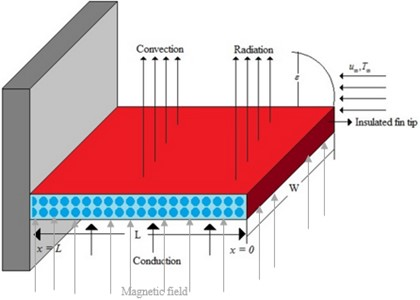The unidirectional energy model based on the assumptions and with the aid of Darcy’s model is:

1
$\frac{d}{d\stackrel{~}{x}}\left(\frac{d\stackrel{~}{T}}{d\stackrel{~}{x}}+\frac{4\sigma }{3{k}_{eff}{\beta }_{R}}\frac{d{\stackrel{~}{T}}^{4}}{d\stackrel{~}{x}}\right)-\frac{\rho \beta {c}_{p}gK}{v{k}_{eff}{A}_{cr}}\left(\stackrel{~}{T}-{T}_{a}{\right)}^{2}-\frac{h\left(1-\epsilon \right)P}{{k}_{eff}{A}_{cr}}\left(\stackrel{~}{T}-{T}_{a}\right)$
$-\frac{\sigma P\in }{{k}_{eff}{A}_{cr}}\left({\stackrel{~}{T}}^{4}-{T}_{a}^{4}\right)-\frac{{\mathbf{J}}_{\mathbf{c}}×{\mathbf{J}}_{\mathbf{c}}}{\sigma {k}_{eff}{A}_{cr}}{A}_{s}=0.$

Eq. (1) can be expressed as:

2
$\frac{{d}^{2}\stackrel{~}{T}}{d{\stackrel{~}{x}}^{2}}+\frac{4\sigma }{3{k}_{eff}{\beta }_{R}}\frac{d}{d\stackrel{~}{x}}\left(\frac{d{\stackrel{~}{T}}^{4}}{d\stackrel{~}{x}}\right)-\frac{\rho \beta {c}_{p}gK}{v\delta {k}_{eff}}\left(\stackrel{~}{T}-{T}_{a}{\right)}^{2}-\frac{h\left(1-\epsilon \right)}{{k}_{eff}\delta }\left(\stackrel{~}{T}-{T}_{a}\right)-\frac{\sigma \in }{{k}_{eff}\delta }\left({\stackrel{~}{T}}^{4}-{T}_{a}^{4}\right)-\frac{{\mathbf{J}}_{\mathbf{c}}×{\mathbf{J}}_{\mathbf{c}}}{\sigma {k}_{eff}{A}_{cr}}{A}_{s}=0.$

The boundary conditions are:

3a
$\stackrel{~}{x}=0,\frac{d\stackrel{~}{T}}{d\stackrel{~}{x}}=0,$
3b
$\stackrel{~}{x}=L,\stackrel{~}{T}={T}_{b}.$

The term ${T}^{4}$ can be expressed as a linear function of temperature as:

4
${\stackrel{~}{T}}^{4}={T}_{a}^{4}+4{T}_{a}^{3}\left(\stackrel{~}{T}-{T}_{a}\right)+6{T}_{a}^{2}{\left(\stackrel{~}{T}-{T}_{\infty }\right)}^{2}+...\cong 4{T}_{a}^{3}\stackrel{~}{T}-3{T}_{a}^{4}.$

Substitution of Eq. (6) into Eq. (5), results in:

5
$\frac{{d}^{2}\stackrel{~}{T}}{d{\stackrel{~}{x}}^{2}}+\frac{16\sigma }{3{k}_{eff}{\beta }_{R}}\frac{{d}^{2}\stackrel{~}{T}}{d{\stackrel{~}{x}}^{2}}-\frac{\rho \beta {c}_{p}gK}{v\delta {k}_{eff}}\left(\stackrel{~}{T}-{T}_{a}{\right)}^{2}-\frac{h\left(1-\epsilon \right)}{{k}_{eff}\delta }\left(\stackrel{~}{T}-{T}_{a}\right)-\frac{4\sigma {T}_{a}^{3}\in }{{k}_{eff}\delta }\left(\stackrel{~}{T}-{T}_{a}\right)-\frac{{\mathbf{J}}_{\mathbf{c}}×{\mathbf{J}}_{\mathbf{c}}}{\sigma {k}_{eff}{A}_{cr}}{A}_{s}=0.$

It should be noted that:

6
$\frac{{\mathbf{J}}_{\mathbf{c}}×{\mathbf{J}}_{\mathbf{c}}}{\sigma }={\sigma }_{m}{B}_{o}^{2}{u}^{2}.$

Therefore:

7
$\frac{{d}^{2}\stackrel{~}{T}}{d{\stackrel{~}{x}}^{2}}+\frac{16\sigma }{3{k}_{eff}{\beta }_{R}}\frac{{d}^{2}\stackrel{~}{T}}{d{\stackrel{~}{x}}^{2}}-\frac{\rho \beta {c}_{p}gK}{v\delta {k}_{eff}}\left(\stackrel{~}{T}-{T}_{a}{\right)}^{2}-\frac{h\left(1-\epsilon \right)}{{k}_{eff}\delta }\left(\stackrel{~}{T}-{T}_{a}\right)$
$-\frac{\sigma {T}_{a}^{3}\in }{{k}_{eff}\delta }\left(\stackrel{~}{T}-{T}_{a}\right)-\frac{{\sigma }_{m\stackrel{~}{T}}{B}_{o}^{2}{u}^{2}}{{A}_{cr}{k}_{eff}}{A}_{s}\left(\stackrel{~}{T}-{T}_{a}\right)=0.$

The magnetic field is taken to be temperature-dependent since the magnetic field varies temperature.

Using the following nondimensional parameters in Eq. (8) on Eqs. (3) and (7):

8
$X=\frac{x}{L},\theta =\frac{T-{T}_{a}}{{T}_{b}-{T}_{a}},Ra=\frac{g\beta k\left({T}_{b}-{T}_{\infty }\right)L}{v\alpha {k}_{r}},{M}^{2}=\frac{hL}{{k}_{eff}t},$
$Rd=\frac{4{\sigma }_{st}{T}_{\infty }^{3}}{3{\beta }_{R}{k}_{eff}},Nr=\frac{4{\sigma }_{st}L{T}_{\infty }^{3}}{{k}_{eff}t},H=\frac{{\sigma }_{m\stackrel{~}{T}}{B}_{o}^{2}{u}^{2}{A}_{s}}{{A}_{cr}{k}_{eff}}.$

The following adimensional form of the governing Eq. (1) is developed:

9
$\frac{{d}^{2}\theta }{d{X}^{2}}+4Rd\frac{{d}^{2}\theta }{d{X}^{2}}-Ra{\theta }^{2}-{M}^{2}\theta -Nr\theta -Ha\theta =0,$

10a
$X=0,\frac{d\theta }{dX}=0,$
10b
$X=1,\theta =1.$

## 3. Application of optimal asymptotic homotopy method

In this section, application of optimal asymptotic homotopy method to the nonlinear model.

For OHAM (developed by Marinca and Herizanu  and  and applied in other works [35-40], we choose the linear operators from Eq. (9) in the form:

11
$L\left[\theta \right]=\frac{{d}^{2}\theta }{d{X}^{2}}.$

The initial approximation ${\theta }_{0}\left(X\right)$ can be obtain as:

12
$\frac{{d}^{2}{\theta }_{0}}{d{X}^{2}}=0,$

with the boundary conditions:

13
$X=0,\frac{d{\theta }_{0}}{dX}=0,\mathrm{}\mathrm{}\mathrm{}\mathrm{}X=1,{\theta }_{0}=1.$

Last equation has solutions:

14
${\theta }_{0}\left(X\right)=1.$

Nonlinear operators corresponding to Eq. (9) and linear operator given in Eq. (14) is defined by:

15
$N\left[\theta \right]=-\alpha {\theta }^{2}\left(X\right)-\beta \theta \left(X\right),$

where:

$\alpha =\frac{Ra}{\left(1+4Rd\right)},\beta =\frac{Nc\left(1-\epsilon \right)+Nr+H}{\left(1+4Rd\right)}.$

By substituting Eq. (14) into Eq. (15), we can obtain the expression of $N\left[{\theta }_{0}\left(X\right)\right]$:

16
$N\left[{\theta }_{0}\left(X\right)\right]=-\alpha -\beta .$

If we consider the first-order approximate solution for nonlinear differential Eq. (9):

17
$\theta \left(X\right)={\theta }_{0}\left(X\right)+{\theta }_{1}\left(X,{C}_{i}\right),$

where ${\theta }_{1}\left(X,{C}_{i}\right)$ are obtained as:

18
$\frac{{d}^{2}{\theta }_{1}}{d{X}^{2}}=N\left[{\theta }_{0}\left(X\right)\right]\hslash \left(X,{C}_{i}\right),$

with boundary conditions:

19
$X=0,\frac{d{\theta }_{1}}{dX}=0,\mathrm{}\mathrm{}\mathrm{}\mathrm{}\mathrm{}X=1,{\theta }_{1}=0.$

Note that the convergence of the approximate solution $\theta \left(X\right)$ depends up on the auxiliary function $\hslash \left(X,{C}_{i}\right)$, we can choose $\hslash \left(X,{C}_{i}\right)$ as:

20
$\hslash \left(X,{C}_{i}\right)={C}_{1}+{C}_{2}{e}^{-X}+{C}_{3}{e}^{-2X}+\cdots {C}_{p}{{e}^{-}}^{\left(p-1\right)X}.$

By solving Eq. (18) with boundary condition Eq. (19), we obtained:

21
${\theta }_{1}\left(X\right)=\frac{1}{2}\left(\beta -\alpha \right)\left\{\frac{2}{9}{C}_{4}\left({e}^{-3X}-{e}^{-3}\right)+\frac{1}{2}{C}_{3}\left({e}^{-2X}-{e}^{-2}\right)+2{C}_{2}\left({e}^{-X}-{e}^{-1}\right)\right\$
$+\left(X-1\right)\left({C}_{1}X+{C}_{1}+2{C}_{2}+{C}_{3}+\frac{2}{3}{C}_{4}\right)}.$

Finally, the solution in Eq. (22) is obtained through Eqs. (14) and (21):

22
$\theta \left(X\right)=1+\frac{1}{2}\left(\beta -\alpha \right)\left\{\frac{2}{9}{C}_{4}\left({e}^{-3X}-{e}^{-3}\right)+\frac{1}{2}{C}_{3}\left({e}^{-2X}-{e}^{-2}\right)+2{C}_{2}\left({e}^{-X}-{e}^{-1}\right)\right\$
$+\left(X-1\right)\left({C}_{1}X+{C}_{1}+2{C}_{2}+{C}_{3}+\frac{2}{3}{C}_{4}\right)},$

where ${C}_{i}$ is unknown parameters which can be obtained with Least-square method (LSM). In our study we choose $p=$4. For example, when $Ra=$0.3, $Rd=$0.4, $Nc=$0.3, $Nr=$0.5, $H=$0.6 and ԑ, the values of constants are: ${C}_{1}=$ –3.034900727, ${C}_{2}=$6.159911506, ${C}_{3}=$ –6.848991138, ${C}_{4}=$2.590773069.

Substituting these values in Eq. (22), we obtain $\theta \left(X\right)$ in a series form as follow:

23
$\theta \left(X\right)=2.652103740-2.463964602{e}^{-X}+0.6848991138{e}^{-2X}$
$-0.1151443183{e}^{-3X}-1.439594147X+0.6069801454{X}^{2}.$

## 4. Efficiency of the fin

The fin efficiency is the ratio of the rate of heat transfer rate by the fin to the rate of heat transfer that would be if the entire fin were at the base temperature. Efficiency of the fin is an indication of thermal performance and is given by:

24
$\eta =\frac{{\int }_{0}^{1}\left[\frac{\rho {c}_{p}gK\beta }{{k}_{eff}tv}\left(T-{T}_{a}{\right)}^{2}+\frac{h}{{k}_{eff}t}\left(T-{T}_{a}\right)+\frac{4\sigma \epsilon {T}_{a}^{3}}{{k}_{eff}t}\left(T-{T}_{a}\right)+\frac{\sigma {B}_{o}^{2}{u}^{2}}{{k}_{eff}{A}_{cr}}\left(T-{T}_{a}\right)\right]dx}{\frac{\rho {c}_{p}gK\beta }{{k}_{eff}tv}\left({T}_{b}-{T}_{a}{\right)}^{2}+\frac{h}{{k}_{eff}t}\left({T}_{b}-{T}_{a}\right)+\frac{4\sigma \epsilon {T}_{a}^{3}}{{k}_{eff}t}\left({T}_{b}-{T}_{a}\right)+\frac{\sigma {B}_{o}^{2}{u}^{2}}{{k}_{eff}{A}_{cr}}\left({T}_{b}-{T}_{a}\right)}.$

Using the dimensional parameters in Eq. (17), we arrived at:

25
$\eta =\frac{Ra{\int }_{0}^{1}{\theta }^{2}dX+\left\{Nc\left(1-\epsilon \right)+Nr+H\right\}{\int }_{0}^{1}\theta dX}{Ra+Nc\left(1-\epsilon \right)+Nr+H}.$

## 5. Results and discussion

The OAHM solutions are simulated for the purpose of graphical illustrations, sensitivity and parametric investigations. Table 1 presents the verifications of results of the OAHM, numerical method (NUM) and differential transformation method (DTM). Although, the DTM provides higher accurate results than OAHM as compared to the results of NM. The higher accuracy is due to the large number of terms (18 terms) in the solutions of DTM as compared to the small number of terms (2 terms). This proves that OAHM is a very convenient mathematical method for the analysis of nonlinear fin thermal models. Also, Table 2 shows the comparison of the results of the present study with the results of the other methods in the previous studies as presented by Patel and Meher . From the results in the table, the validity and superiority of the optimal homotopy asymptotic method are established as the method presents better results with the results of the numerical method.

The significance of various parameters of the nonlinear model on the thermal management enhancement of thermal systems using the solutions presented are graphical represented for pictorial discussion in Figs. 2-11. The results illustrate that the augmentations of the conductive-radiative, conductive-convective, porosity and magnetic field cause the extended surface adimensional temperature to reduce as a result of increased rate of heat flow via the passive device. The graphical illustrations show that the efficiency and effectiveness of the fin is high at low values of the radiative-conductive, convective-conductive, porosity and magnetic field parameters.

Table 1Comparative of results methods using OAHM, DTM and NUM for θX when Rd= 0.5, ε= 0.1, Ra= 0.4, Nc= 0.3, Nr= 0.2, H= 0.1

 X NUM  DTM Ordre 18  OAHM Ordre 1 Difference 1 Difference 2 0.0 0.86349923 0.86349915 0.86349987 0.00000008 0.00000064 0.1 0.86481708 0.86481703 0.86481420 0.00000005 0.00000288 0.2 0.86877626 0.86877619 0.86877319 0.00000007 0.00000307 0.3 0.87539340 0.87539333 0.87539383 0.00000007 0.00000043 0.4 0.88469650 0.88469643 0.88469975 0.00000007 0.00000325 0.5 0.89672509 0.89672504 0.89672757 0.00000005 0.00000248 0.6 0.91153065 0.91153060 0.91152929 0.00000005 0.00000136 0.7 0.92917705 0.92917701 0.92917206 0.00000004 0.00000499 0.8 0.94974120 0.94974116 0.94973660 0.00000004 0.00000460 0.9 0.97331376 0.97331372 0.97331485 0.00000004 0.00000109 1.0 1.00000000 1.00000000 1.00000741 0.00000000 0.00000741 Difference 1$\mathrm{}=│{\theta }_{NUM}–{\theta }_{DTM}│$, Difference 2 $=│{\theta }_{NUM}–{\theta }_{OHAM}│$

Table 2Comparative of the results of methods using OHAM, ADSTM and LSM Nr= 0.3, Nc= 0.4,H= 0.9, Rd= 0.5 and Ra= 0.1

 X NUM ADSTM  LSM  OAHM 0.0 0.781820729 0.781820594 0.781820569 0.781820657 0.1 0.783904154 0.783904049 0.783904760 0.783904121 0.2 0.790166187 0.790166063 0.790165668 0.790166132 0.3 0.800641805 0.800641676 0.800641589 0.800641734 0.4 0.815389668 0.815389550 0.815389906 0.815389592 0.5 0.834492509 0.834492402 0.834492969 0.834492478 0.6 0.858057690 0.858057590 0.858057970 0.858057623 0.7 0.886217964 0.886217887 0.886217828 0.886217919 0.8 0.919132513 0.919132442 0.919132060 0.919132480 0.9 0.956988020 0.956987943 0.956987665 0.956987985 1.0 1.000000000 1.000000000 1.000000000 1.000000000

The impacts of convective-conductive, radiative-conductive and porosity parameters on the adimensional temperature distribution in the passive device is graphically illustrated in Fig. 2. The figure shows that as the convection-radiative increases the adimensional temperature in the fin increases. This also means that the local temperature in the extended surface increases as the conduction-convection parameter increases.

Fig. 2Effects of conductive-radiative on the temperature distribution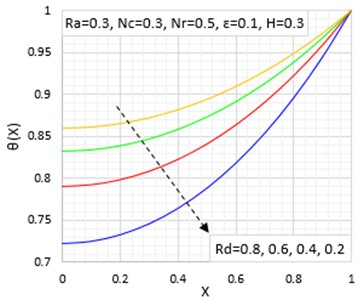Fig. 3Effects of porous parameter on the temperature distribution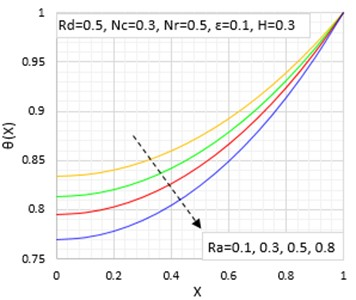It is presented in Fig. 3 about the impact of porosity on the extended surface temperature behaviour. The graphical illustrations show that the amplification of parameter of porosity (Rayleigh number) causes the passive device temperature to be lessened because of the increased permeability allowed by the fin.

Figs. 4 and 5 display the effects of convective-conductive and radiative-conductive parameters on the fin temperature behaviour. It is shown that the rise of the conductive-radiative, and conductive-convective cause the extended surface adimensional temperature to fall as a result of increased rate of heat flow via the fin. The graphical illustrations show that the efficiency and effectiveness of the fin is high at low values of the radiative-conductive, convective-conductive, porosity and magnetic field parameters.

Fig. 4Effects of convective parameter on the temperature distribution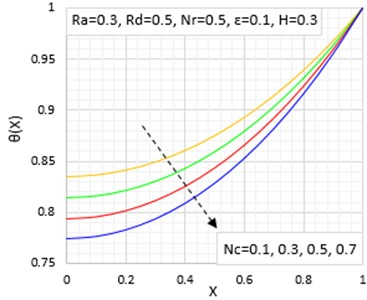Fig. 5Effects of radiative parameter on the temperature distribution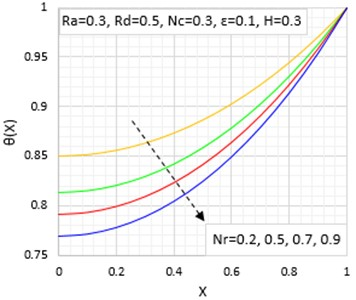Fig. 6Effects of magnetic parameter on the temperature distribution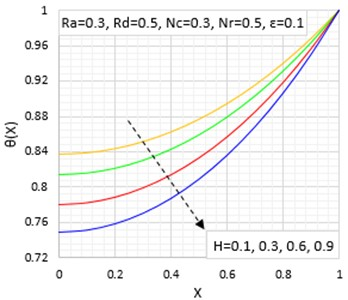Fig. 7Effects of porosity/void ratio on the temperature distribution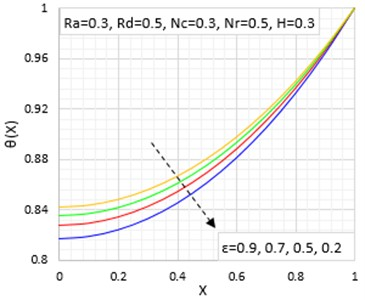Fig. 6 displays the effect of value of magnetic field or Lorentz force on the fin temperature behaviours. It is illustrated that when the value of the parameter of the magnetic field increase, the passive device temperature decrease. The graphical illustrations show that the efficiency and effectiveness of the fin is high at low values of the magnetic field parameters.

The effects of convective-conductive, radiative-conductive, magnetic field and porous parameters on the thermal efficiency of the fin are presented in Figs. 8, 9 and 10 while the effect of porosity or void ratio on the fin thermal efficiency is shown in Fig. 11. It is shown in the figures that when the convective-conductive, radiative-conductive, porosity and magnetic field parameters rise, the passive device efficiency falls.

Fig. 8Effects of convective parameter on the thermal efficiency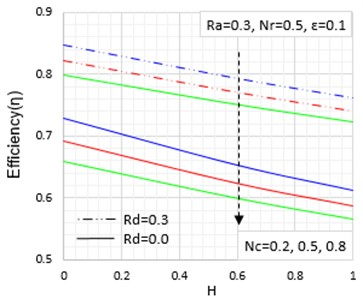Fig. 9Effects of radiative parameter on the thermal efficiency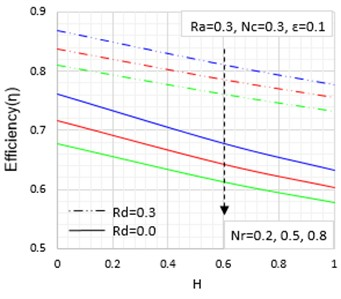Fig. 10Effects of porous parameter on the temperature distribution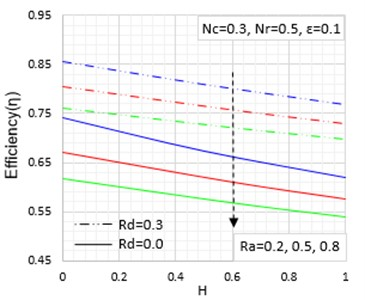Fig. 11Effects of porosity/void ratio on the temperature distribution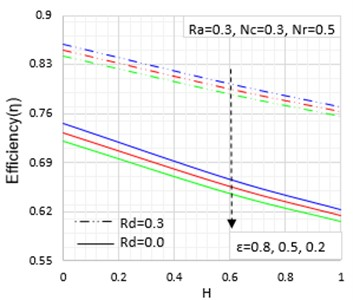## 6. Conclusions

In this work, optimal asymptotic homotopy method has been used to investigate the heat transfer characteristics of a convective-radiative porous fin with temperature-invariant thermal conductivity. The effect of various parameters of the nonlinear model on the thermal management enhancement of thermal systems have been explored using the solutions presented by the approximate analytical method. The graphical representations of the thermal behaviour of the extended surfaces have been presented and the results have been discussed. The study has showed that the augmentations of the conductive-radiative, conductive-convective, porosity and magnetic field cause the extended surface temperature to reduce as a result of increased rate of heat flow via the passive device. The graphical illustrations show that the efficiency and effectiveness of the fin is high at low values of the radiative-conductive, convective-conductive, porosity and magnetic field parameters. This study will assist in proper thermal analysis of fins and will help in the passive device design.

28 September 2022
Accepted
13 September 2023
Published
17 November 2023
Keywords
fins
thermal analysis
optimal homotopy asymptotic method
thermal performance
Acknowledgements

The authors have not disclosed any funding.

Data Availability

The datasets generated during and/or analyzed during the current study are available from the corresponding author on reasonable request.

Author Contributions

Sobamowo M. Gbeminiyi: ideas and formulation of the research. model development. Writing – original draft preparation. writing – review and editing. application of numerical method to analyze the problem.

Hamza Berrehal: Provided solutions, simulated the solutions, Provided data and application of both analytical and numerical methods to analyse the problem.

Arinola Bola Ajayi: verification of the overall replication/reproducibility of results. writing of original draft preparation.

Olutayo Onanuga: writing of the paper – review and editing. verification of the overall replication/reproducibility of results.

Rotimi Fawumi : provisions of resources, verification of the overall replication/reproducibility of results. writing of original draft preparation.

Conflict of interest

The authors declare that they have no conflict of interest.